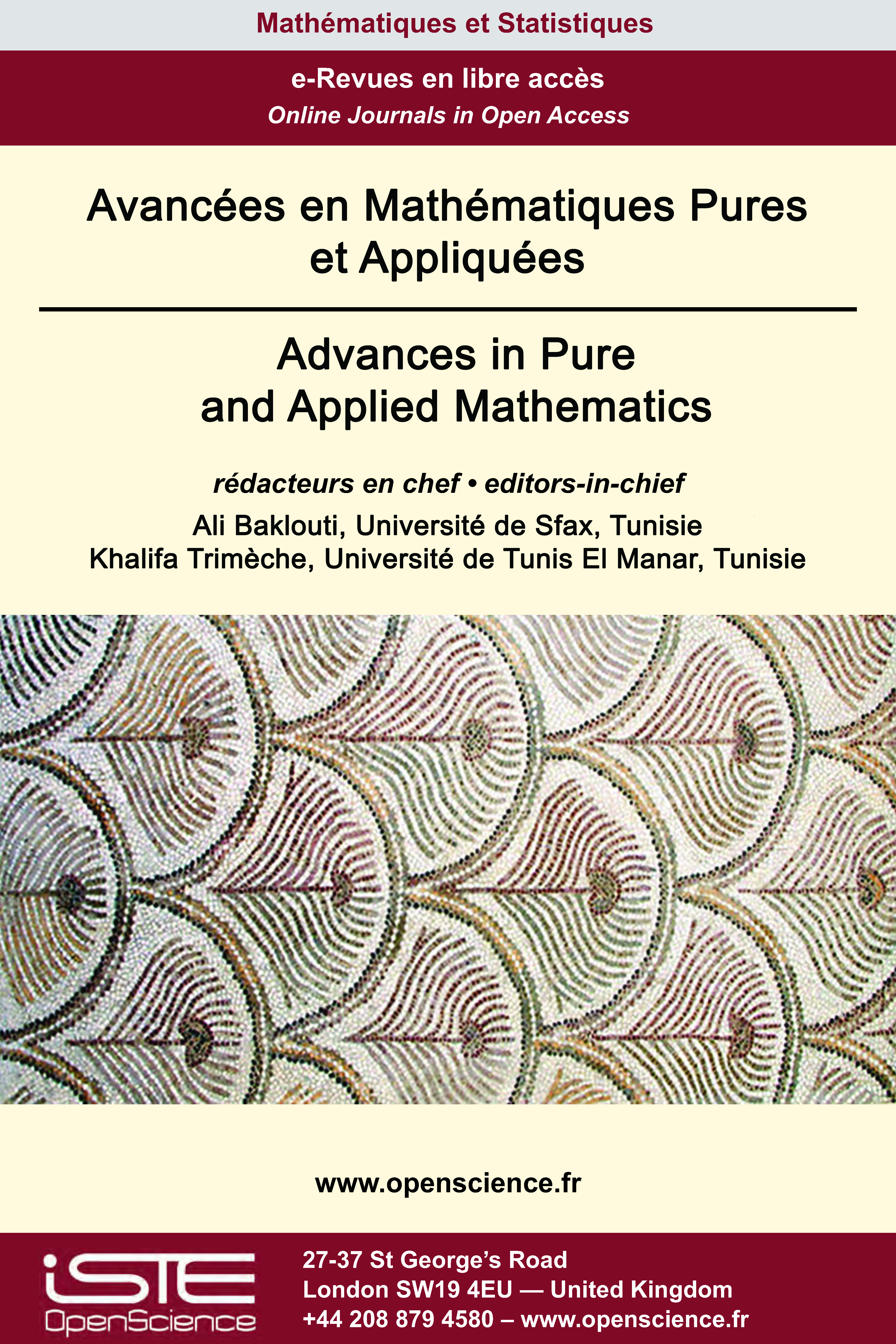# Vol 11 - Issue 1 (May 2020)

## List of Articles

Regular Solutions for the relativistic Boltzmann equation in Yang-Mills field

We consider in this work the Boltzmann equation in the presence of a Yang-Mills fields in temporal gauge, which generalizes to the non-Abelian case the electromagnetic field. We prove, using the method presented by N. Noutchegueme and R. D. Ayissi , a local in time existence and uniqueness theorem for the regular solutions.

A new characterization of commutative semiregular rings

A commutative ring R is called J-rad clean in case, for any r ∈ R, there is an idempotent e ∈ R such that r−e ∈ U(R) and re ∈ J(R), where U(R) and J(R) denote the set of units and the Jacobson radical of R, respectively. Also, a ring R is called semiregular if R/J(R) is regular in the sense of von Neumann and idempotents lift modulo J(R). We demonstrate that these two concepts are, actually, equivalent and we portray a portion of the properties of this class of rings. In particular, as a direct application, we prove that the commutative group ring RG is J-rad clean if, and only if, R is a commutative J-rad clean ring and G is a torsion abelian group, provided that J(R) is nil.

Products of Complex Rectangular and Hermitian Random Matrices

Very recently, it has been shown for products of real matrices with anti-symmetric matrices of even dimension that the traditional harmonic analysis on matrix groups developed by Harish-Chandra et al. needs to be modified when considering the group action on general symmetric spaces of matrices. In the present work, we consider the product of complex random matrices with Hermitian matrices, in particular the former can be also rectangular while the latter has not to be positive definite and is considered as a fixed matrix as well as a random matrix. This generalises an approach for products involving the Gaussian unitary ensemble (GUE) and circumvents the use there of non-compact group integrals. We derive the joint probability density function of the real eigenvalues and, additionally, prove transformation formulas for the bi-orthogonal functions and kernels.

∗-Lie higher derivable mappings on ∗-rings

In this paper, it is shown that, if R is a ∗-ring containing a nontrivial self adjoint idempotent which admits a ∗-Lie higher derivable mapping L={Ln}n∈N, then there exists an element ZX,Y (depending on X and Y) in the center Z(R) such that Ln(X+Y)=Ln(X)+Ln(Y)+ZX,Y.### Other issues :

2020

Volume 20- 11

Issue 1 (May 2020)
Issue 2 (September 2020)

Forthcoming papers